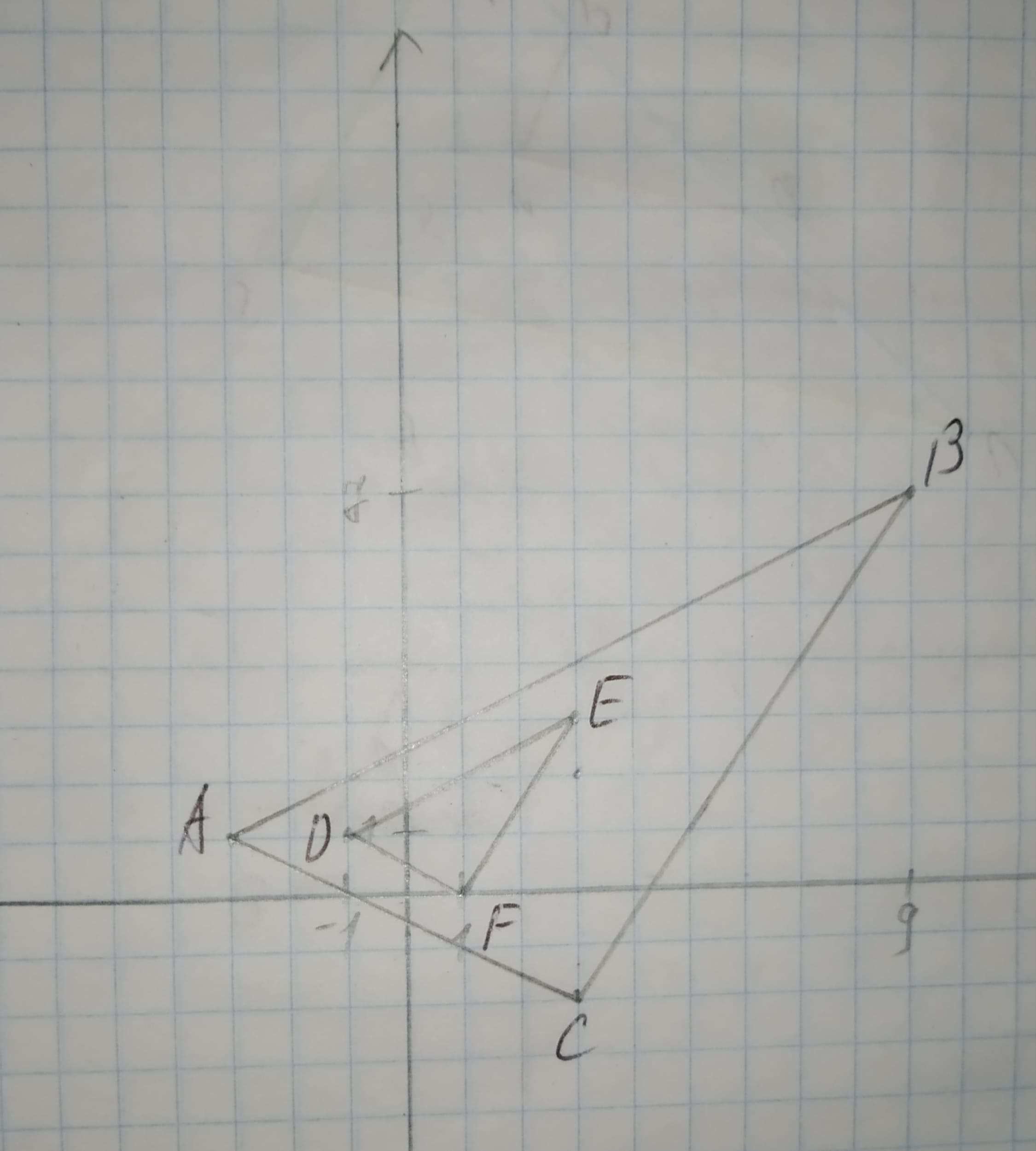Question# To sketch:The graph from the points and verify that the dilation is a similarity transformation. Given information: A(-3,1),B(9,7),C(3,2),D(-1,1)E(3,3),F(1,0).

Similarity
ANSWEREDTo sketch:The graph from the points and verify that the dilation is a similarity transformation.
Given information:
A(-3,1),B(9,7),C(3,2),D(-1,1)E(3,3),F(1,0).2020-12-18

Graph,
Consider the given points A(-3,1),B(9,7),C(3,2),D(-1,1)E(3,3),F(1,0).
Now, draw the graph.Interpretation:
From the figure there are two triangles $$\displaystyle\triangle{A}{B}{C}{\quad\text{and}\quad}\triangle{E}{D}{F}$$.
Now,
$$\displaystyle\frac{{{A}{B}}}{{{D}{E}}}=\frac{{{B}{C}}}{{{E}{F}}}=\frac{{{A}{C}}}{{{D}{F}}}=\frac{{3}}{{1}}$$
Thus, by SSS similarity theorem triangles are similar.